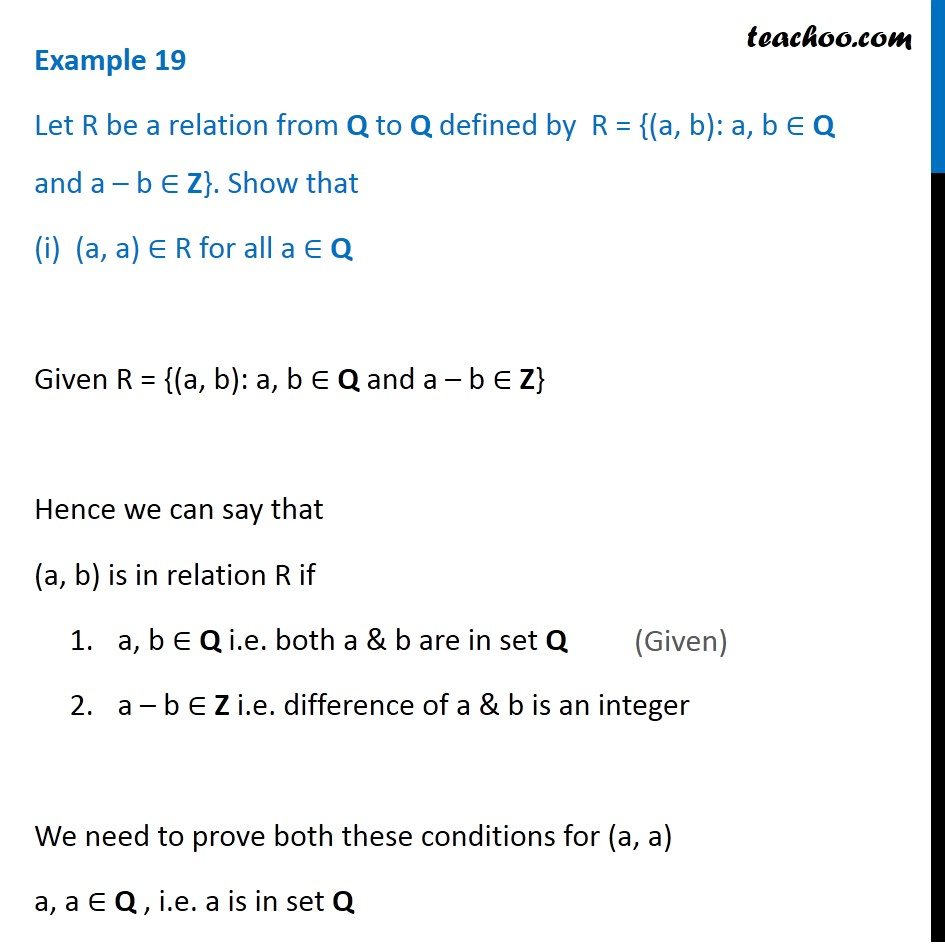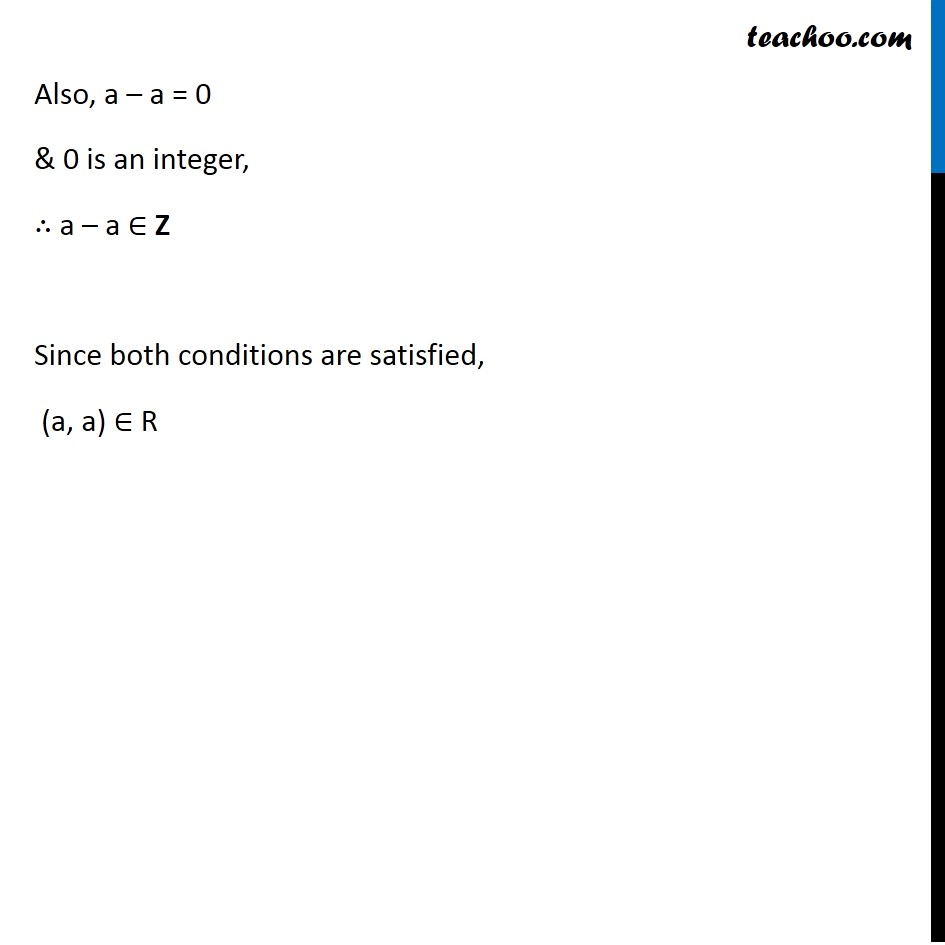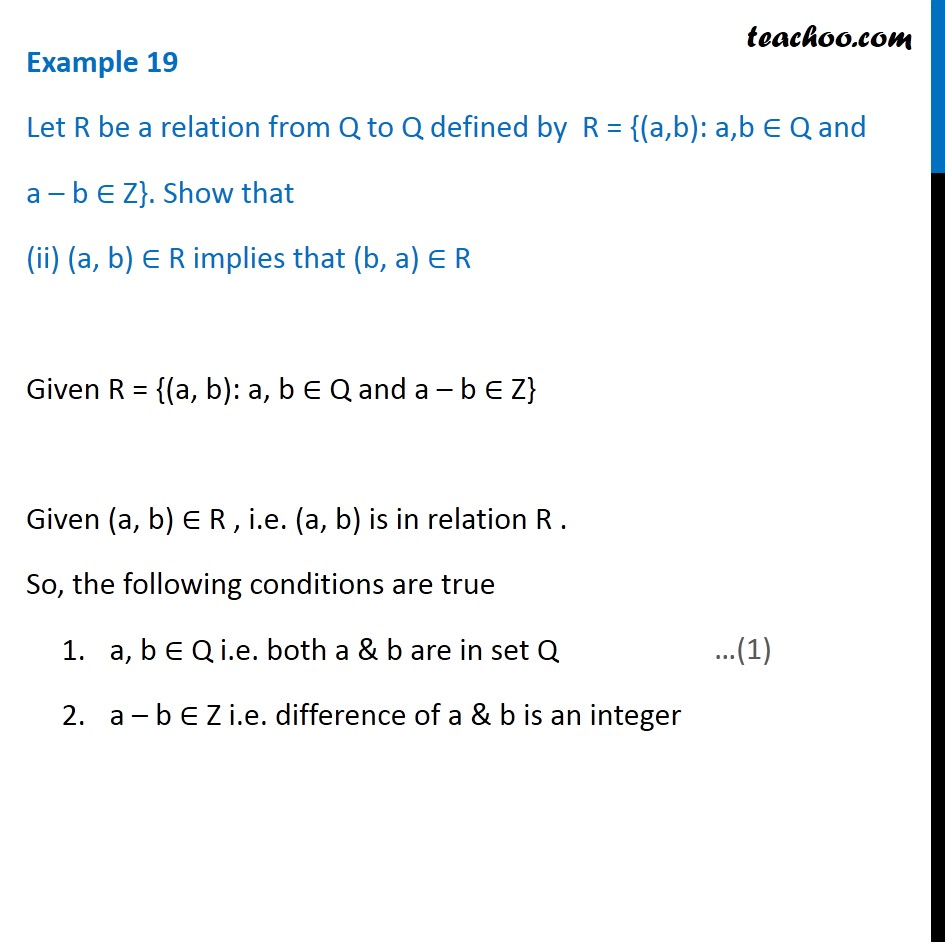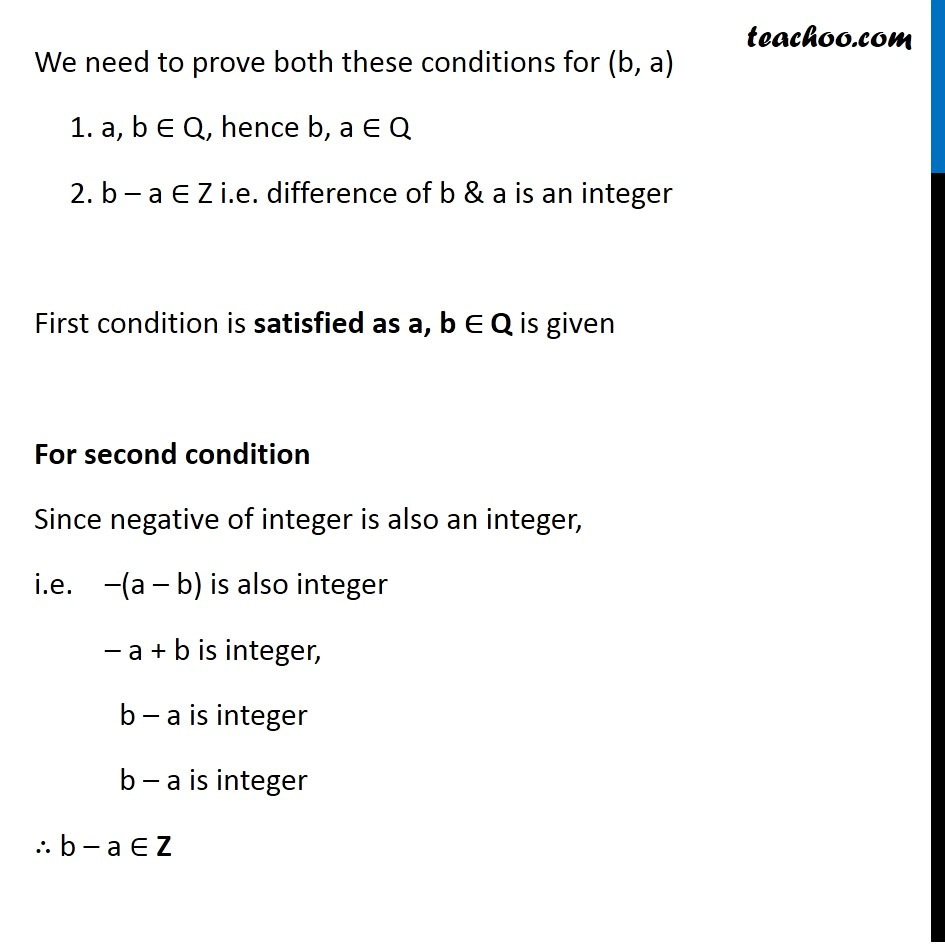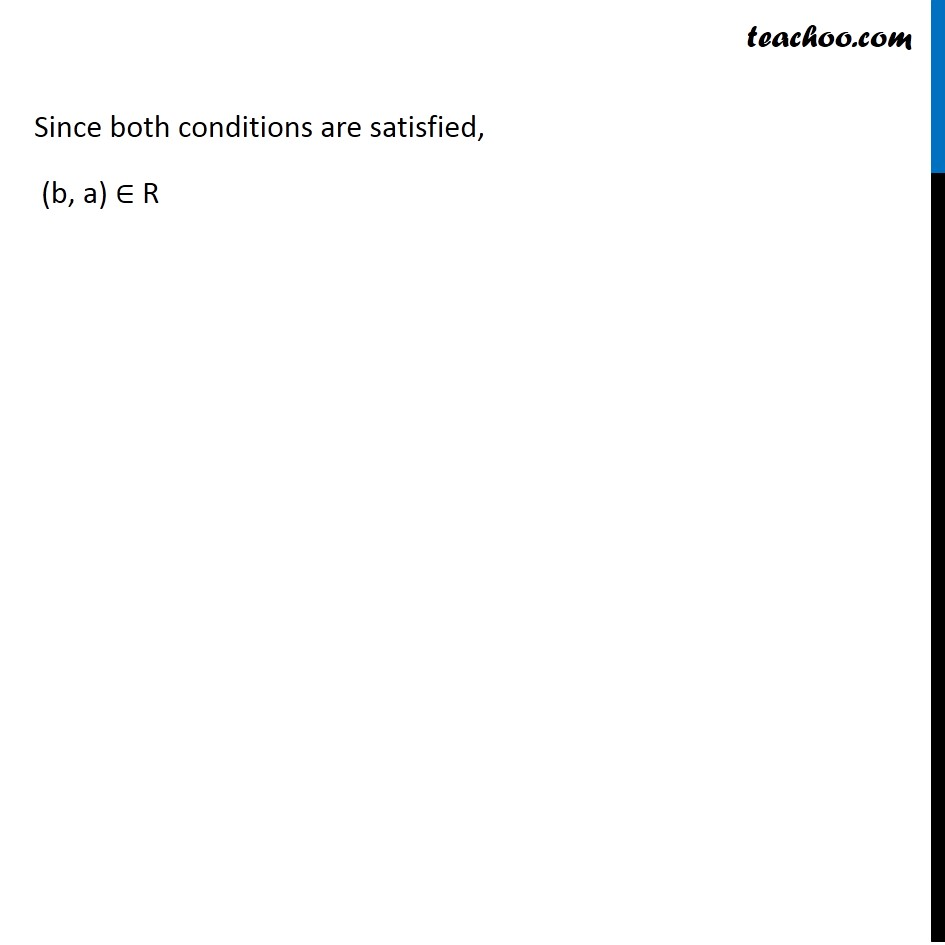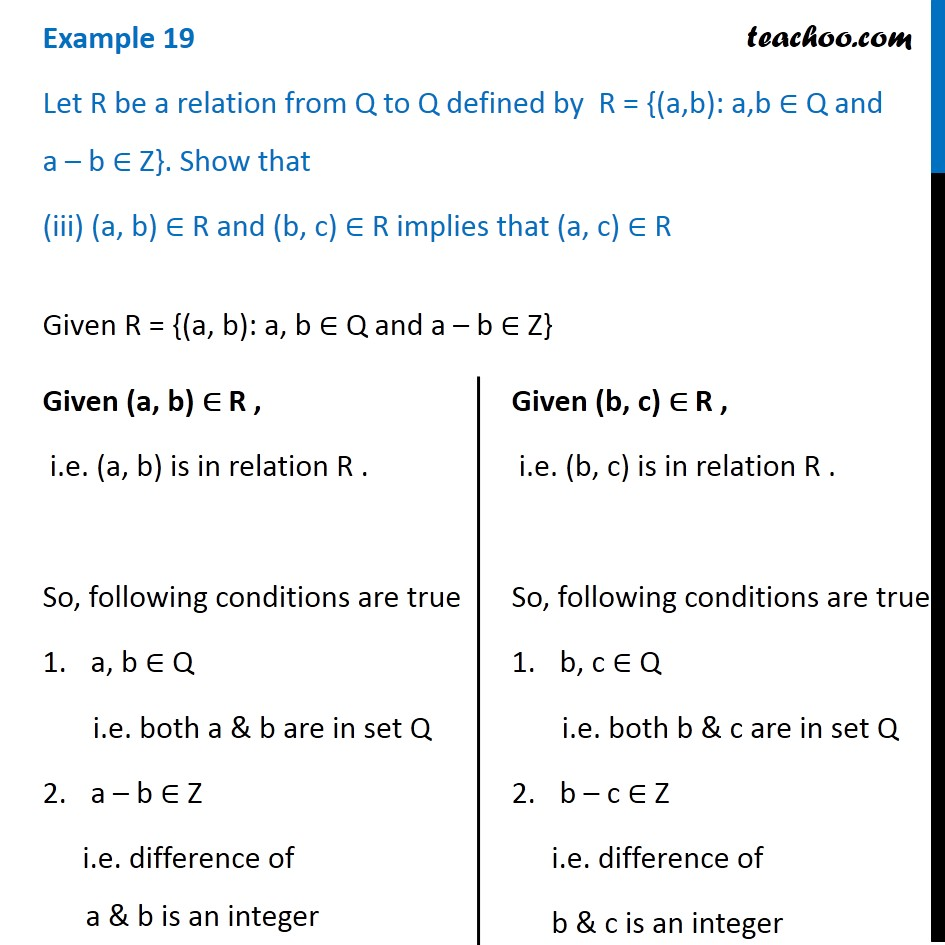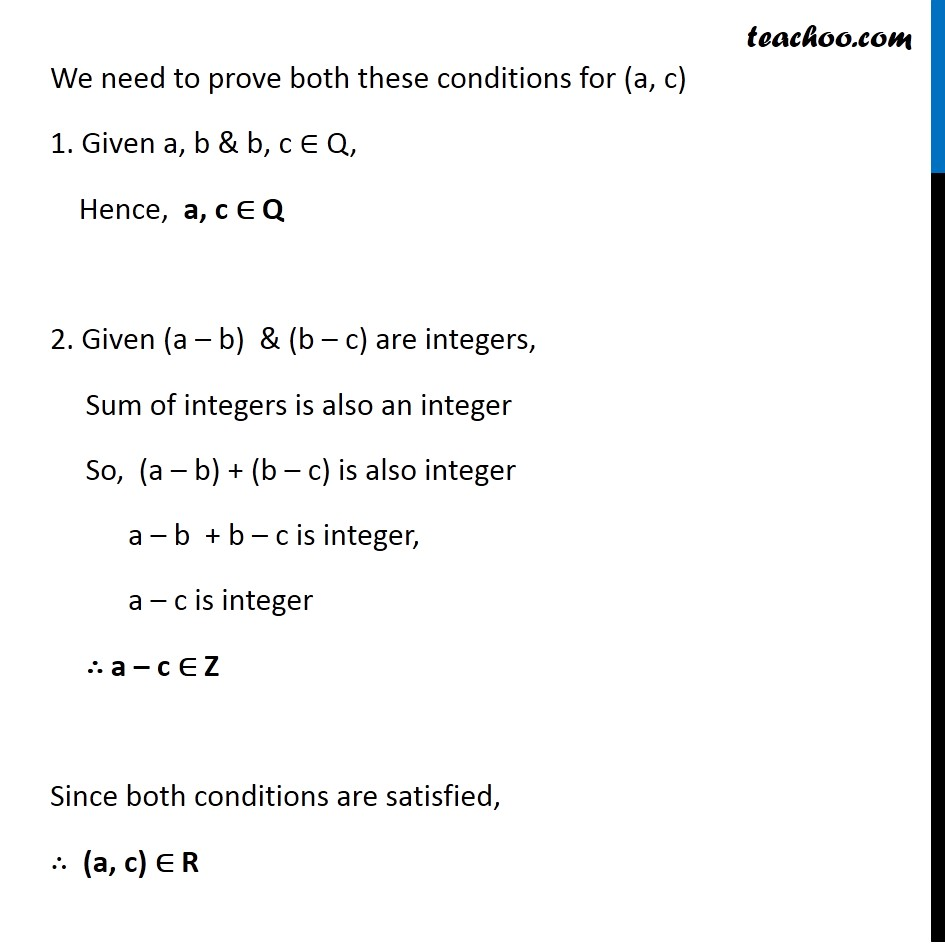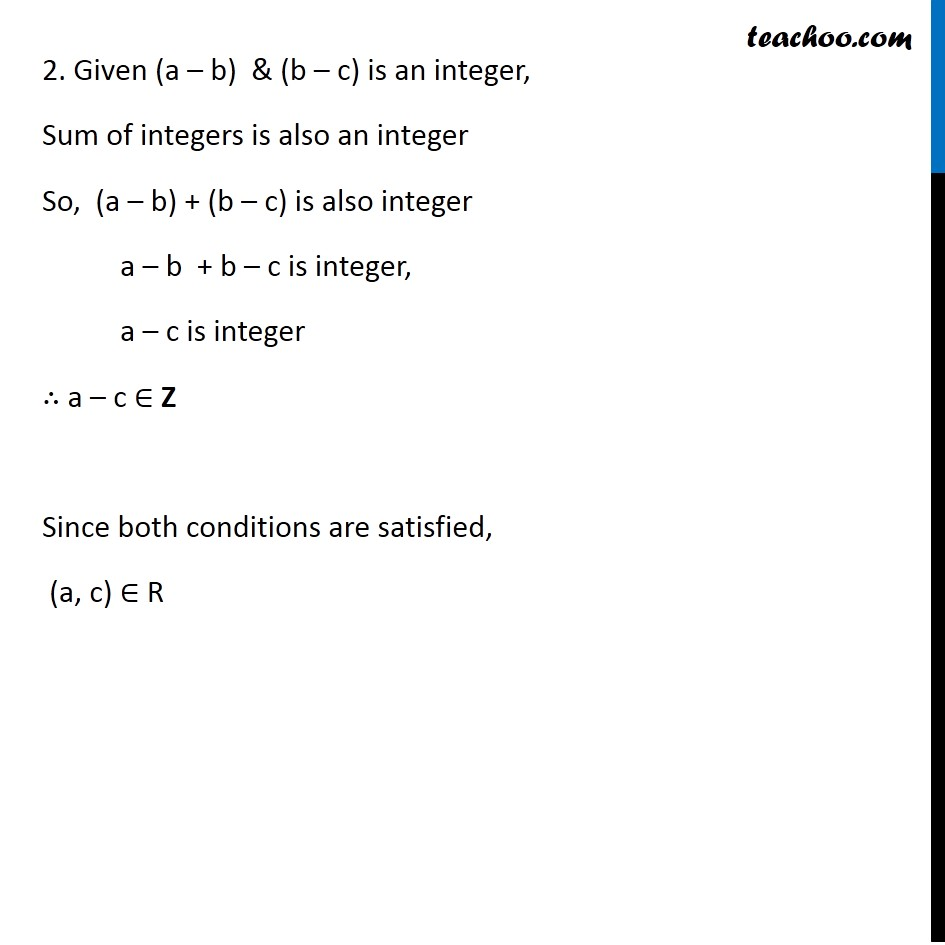1. Chapter 2 Class 11 Relations and Functions
2. Concept wise
3. Relations - Definition

Transcript

Example 19 Let R be a relation from Q to Q defined by R = {(a, b): a, b ∈ Q and a – b ∈ Z}. Show that (i) (a, a) ∈ R for all a ∈ Q Given R = {(a, b): a, b ∈ Q and a – b ∈ Z} Hence we can say that (a, b) is in relation R if a, b ∈ Q i.e. both a & b are in set Q a – b ∈ Z i.e. difference of a & b is an integer We need to prove both these conditions for (a, a) a, a ∈ Q , i.e. a is in set Q Example 19 Let R be a relation from Q to Q defined by R = {(a, b): a, b ∈ Q and a – b ∈ Z}. Show that (i) (a, a) ∈ R for all a ∈ Q Given R = {(a, b): a, b ∈ Q and a – b ∈ Z} Hence we can say that (a, b) is in relation R if a, b ∈ Q i.e. both a & b are in set Q a – b ∈ Z i.e. difference of a & b is an integer We need to prove both these conditions for (a, a) a, a ∈ Q , i.e. a is in set Q Also, a – a = 0 & 0 is an integer, ∴ a – a ∈ Z Since both conditions are satisfied, (a, a) ∈ R Example 19 Let R be a relation from Q to Q defined by R = {(a,b): a,b ∈ Q and a – b ∈ Z}. Show that (ii) (a, b) ∈ R implies that (b, a) ∈ R Given R = {(a, b): a, b ∈ Q and a – b ∈ Z} Given (a, b) ∈ R , i.e. (a, b) is in relation R . So, the following conditions are true a, b ∈ Q i.e. both a & b are in set Q a – b ∈ Z i.e. difference of a & b is an integer We need to prove both these conditions for (b, a) 1. a, b ∈ Q, hence b, a ∈ Q 2. b – a ∈ Z i.e. difference of b & a is an integer First condition is satisfied as a, b ∈ Q is given For second condition Since negative of integer is also an integer, i.e. –(a – b) is also integer – a + b is integer, b – a is integer b – a is integer ∴ b – a ∈ Z Since both conditions are satisfied, (b, a) ∈ R Example 19 Let R be a relation from Q to Q defined by R = {(a,b): a,b ∈ Q and a – b ∈ Z}. Show that (iii) (a, b) ∈ R and (b, c) ∈ R implies that (a, c) ∈ R Given R = {(a, b): a, b ∈ Q and a – b ∈ Z} Given (a, b) ∈ R , i.e. (a, b) is in relation R . So, following conditions are true a, b ∈ Q i.e. both a & b are in set Q a – b ∈ Z i.e. difference of a & b is an integer Given (b, c) ∈ R , i.e. (b, c) is in relation R . So, following conditions are true b, c ∈ Q i.e. both b & c are in set Q b – c ∈ Z i.e. difference of b & c is an integer We need to prove both these conditions for (a, c) 1. Given a, b & b, c ∈ Q, Hence, a, c ∈ Q 2. Given (a – b) & (b – c) are integers, Sum of integers is also an integer So, (a – b) + (b – c) is also integer a – b + b – c is integer, a – c is integer ∴ a – c ∈ Z Since both conditions are satisfied, ∴ (a, c) ∈ R 2. Given (a – b) & (b – c) is an integer, Sum of integers is also an integer So, (a – b) + (b – c) is also integer a – b + b – c is integer, a – c is integer ∴ a – c ∈ Z Since both conditions are satisfied, (a, c) ∈ R

Relations - Definition